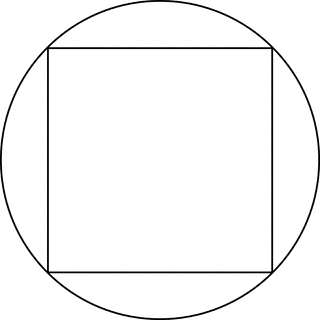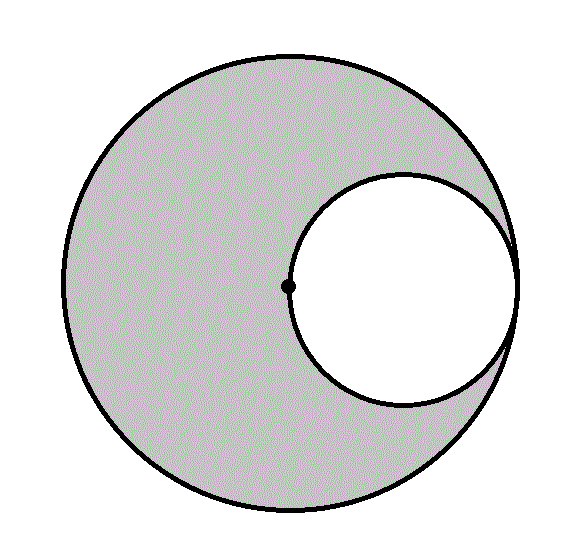# Quantitative Geometry

This is an MCQ quiz for GMAT, which includes questions on quantitative reasoning (geometry).

Start QuizSquare S is inscribed in circle C as in the figure above. What is the circumference of C?
(1) The perimeter of S is 16.
(2) The area of S is 36.

EACH statement ALONE is sufficient.

Statement (2) ALONE is sufficient, but statement (1) alone is not sufficient.

BOTH statements TOGETHER are sufficient, but NEITHER statement ALONE is sufficient.

Statement (1) ALONE is sufficient, but statement (2) alone is not sufficient.

Statements (1) and (2) TOGETHER are NOT sufficient.

What is the circumference of the circle given by:

(x−7)2+(y−14)2=r2

I) r2=225.

II) The slope of the tangent to the circle at (22,14) is undefined.

Statement II is sufficient to answer the question, but Statement I is not sufficient to answer the question.

Statement I is sufficient to answer the question, but Statement II is not sufficient to answer the question.

Both statements together are needed to answer the question.

Either statement alone is sufficient to answer the question.

What is the circumference of Circle P?

1.) The radius of the circle is 9.

2.) The circle is inside another circle of area 81π.

Statement 2 is sufficient to solve the question, but Statement 1 is not sufficient to solve the question.

Statement 1 is sufficient to solve the question, but Statement 2 is not sufficient to solve the question.

Each statement alone is sufficient to solve the question.

Both statements taken together are sufficient to solve the question.

Neither statement is sufficient to solve the question. More information is needed.

Data Sufficiency Question

Calculate the area of a circle.
1. The radius of the circle is 4.
2. The circumference of the circle is 24.

Each statement alone is sufficient

Statement 2 alone is sufficient, but statement 1 alone is not sufficient to answer the question

Statements 1 and 2 together are not sufficient, and additional data is needed to answer the question

Statement 1 alone is sufficient, but statement 2 alone is not sufficient to answer the question

Both statements taken together are sufficient to answer the question, but neither statement alone is sufficientWhat is the area of the gray region in the above figure?
Statement 1: The diameter of the larger circle is one mile.
Statement 2: The radius of the smaller circle is 1,320 feet.

BOTH statements TOGETHER are sufficient to answer the question, but NEITHER statement ALONE is sufficient to answer the question.

EITHER statement ALONE is sufficient to answer the question.

BOTH statements TOGETHER are insufficient to answer the question.

Statement 1 ALONE is sufficient to answer the question, but Statement 2 ALONE is NOT sufficient to answer the question.

Statement 2 ALONE is sufficient to answer the question, but Statement 1 ALONE is NOT sufficient to answer the question.

Two circles are constructed; one is inscribed inside a given regular hexagon, and the other is circumscribed about the same hexagon.

What is the area of the inscribed circle?

Statement 1: The area of the circumscribed circle is 25π

Statement 2: The perimeter of the hexagon is 30.

Statement 1 ALONE is sufficient to answer the question, but Statement 2 ALONE is NOT sufficient to answer the question.

Statement 2 ALONE is sufficient to answer the question, but Statement 1 ALONE is NOT sufficient to answer the question.

EITHER statement ALONE is sufficient to answer the question.

BOTH statements TOGETHER are insufficient to answer the question.

BOTH statements TOGETHER are sufficient to answer the question, but NEITHER statement ALONE is sufficient to answer the question.

You are given a circle and a square. Which one has the larger area?

Statement 1: The radius of the circle is two-thirds the sidelength of the square.

Statement 2: The circumference of the circle is π3 times the perimeter of the square.

BOTH statements TOGETHER are insufficient to answer the question.

EITHER statement ALONE is sufficient to answer the question.

Statement 2 ALONE is sufficient to answer the question, but Statement 1 ALONE is NOT sufficient to answer the question

Statement 1 ALONE is sufficient to answer the question, but Statement 2 ALONE is NOT sufficient to answer the question.

BOTH statements TOGETHER are sufficient to answer the question, but NEITHER statement ALONE is sufficient to answer the question.

You are given a circle and an equilateral triangle. Which one has the greater area?
Statement 1: The sidelength of the triangle is three times the radius of the circle.
Statement 2: The perimeter of the triangle is 99 inches.

Statement 2 ALONE is sufficient to answer the question, but Statement 1 ALONE is NOT sufficient to answer the question.

BOTH statements TOGETHER are insufficient to answer the question.

Statement 1 ALONE is sufficient to answer the question, but Statement 2 ALONE is NOT sufficient to answer the question.

BOTH statements TOGETHER are sufficient to answer the question, but NEITHER statement ALONE is sufficient to answer the question.

EITHER statement ALONE is sufficient to answer the question.

What is the circumference of circle J?

I) Circle J has an area of 64πin2.

II) Circle J has a diameter of 16in.

Statement 2 is sufficient to solve the question, but statement 1 is not sufficient to solve the question.

Both statements taken together are sufficient to solve the question.

Each statement alone is enough to solve the question.

Statement 1 is sufficient to solve the question, but statement 2 is not sufficient to solve the question.

Neither statement is sufficient to solve the question. More information is needed.

What is the circumference of Circle V?

1.) The diameter of the circle is 10.

2.) The area of the circle is 25π.

Statement 1 is sufficient to solve the question, but Statement 2 is not sufficient to solve the question.

Neither statement is sufficient to solve the question. More information is needed.

Each statement alone is sufficient to solve the question.

Statement 2 is sufficient to solve the question, but Statement 1 is not sufficient to solve the question.

Both statements taken together are sufficient to solve the question.

Quiz/Test Summary
Title: Quantitative Geometry
Questions: 10
Contributed by: